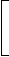# Data Interpretation - Pie Charts - Discussion

Discussion Forum : Pie Charts - Pie Chart 12 (Q.No. 1)
Directions to Solve

Study the following graph and the table and answer the questions given below.

Data of different states regarding population of states in the year 1998Total population of the given States = 3276000.

 States Sex and Literacy wise Population Ratio Sex Literacy M F Literate Illiterate A.P 5 3 2 7 M.P 3 1 1 4 Delhi 2 3 2 1 Goa 3 5 3 2 Bihar 3 4 4 1 U.P. 3 2 7 2 T.N. 3 4 9 4

1.
What will be the percentage of total number of males in U.P., M.P. and Goa together to the total population of all the given states?
25%
27.5%
28.5%
31.5%
Explanation:

 Number of males in U.P =3 of (15% of N)= 3 x 15 x N   = 9 x N . 5 5 100 100

where N = 3276000.

 Number of males in M.P =3 of (20% of N)= 3 x 20 x N   = 15 x N . 4 4 100 100

 Number of males in Goa =3 of (12% of N)= 3 x 12 x N   = 4.5 x N . 8 8 100 100Total number of males in these three states
 = (9 + 15 + 4.5) x N 100
 =28.5 x N. 100Required Percentage =28.5 x N100
x 100% = 28.5%.
N

Discussion:
15 comments Page 1 of 2.

Thinley Gyeltshen said:   3 years ago
First, find a total number of males.

UP = 3/5 * (15% of 3276000) = 294840
MP = 3/4 * (20% of 3276000) = 491400
GOA = 3/8 * (12% of 3276000) = 147420

Now % = total no. Of males(UP + MP + GOA) divide by 3276000* 100.
= 28.5%.
(3)

Ugyen said:   5 years ago
Why we have to add 3+2 in the first step?
(2)

Jimmy said:   5 years ago
How come a number of people in decimals, Like 4.5?

Riri said:   5 years ago
Here They have taken the whole ratio of the population of M +F.

Anonymous said:   6 years ago
In the question they didn't mention that the men and female are in the ratio.
(1)

Akhilesh said:   7 years ago
What is the average score of this test? Please tell me the derivation part.

Lisa said:   8 years ago
How do you get the answers of 9, 14 and 4.5?

Rithish said:   8 years ago
U.P (Male, Female ratio) = 3 : 2 = 3/(3 + 2) = 3/5.

M.P = 3:1 = 3/4.

Goa = 3:5 = 3/8.

We know that 3276000 value remains same for all states.

So, take only the percentages and assume 3276000 as 'N'.

U.P = Population percentage = 15%.

M.P = 20%.

Goa = 12%.

No of men in U.P = 3/5 (15/100) N = (9/100) N.

M.P = 3/4 (20/100) N = (15/100) N.

Goa = 3/8 (12/100) N = (4.5/100) N.

Adding these three, we get (28.5/100) N.

Required % = {(28.5/100)N/N } * 100 = 28.5%.

Raquel said:   8 years ago
Does any one have a simpler solution to this problem? I don't understand the working out.

Sai said:   9 years ago
Can anyone tell me how did come 15%? I cannot understand this problem?